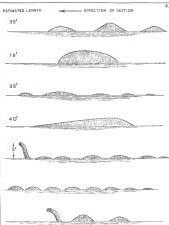#### You may also like### Degree Ceremony

What does Pythagoras' Theorem tell you about these angles: 90Â°, (45+x)Â° and (45-x)Â° in a triangle?### After Thought

Which is larger cos(sin x) or sin(cos x) ? Does this depend on x ?### Small Steps

Two problems about infinite processes where smaller and smaller steps are taken and you have to discover what happens in the limit.

# Loch Ness

##### Age 16 to 18Challenge Level(1) Plot the graph of the function $y=f(x)$ where $f(x) = \sin x +|\sin x|$. Find the first derivative of this function and say where it is defined and where it is not defined. (2) Express the function $f(x) = \sin x + \cos x$ in the form $f(x)=A\sin (x+\alpha)$, find $A$ and $\alpha$ and plot the graph of this function. Similarly express the function $g(x) = \sin x - \cos x$ in the form $g(x) = B\sin (x +\beta)$ where $-\pi /2 < \beta < \pi /2$, and plot its graph on the same axes. (3) Plot the graph of the function $y=f(x)$ where $f(x)= \sin x + |\cos x|$. Find the first derivative of this function and say where it is defined and where it is not defined.

NOTES AND BACKGROUND
These graphs are not monsters. They are humpy because the functions are periodic and involve sines, cosines and absolute values. This problem calls for you to describe and explain the features of the graphs.

First think about the features of the graphs and try to sketch them for yourself, then it may help to use a graphic calculator or graphing software.

Andrei from Romania chose this problem for the NRICH 10th Anniversary special collection. He said "The problems you are posting on the site are usually, at least for me, far from the problems we solve in school or in other activities related to school."

We would like to hear from other students. Is this your experience?

Andrei says "It's a difficult task to choose the problems I liked most, and to choose one seems impossible." So he also chose Over-booking and Wobbler.